CBSE Class 12 Sample Paper for 2022 Boards (For Term 2)

Class 12
Solutions of Sample Papers and Past Year Papers - for Class 12 Boards

## Find the foot of the perpendicular from the point (1, 2, 0) upon the plane x – 3y + 2z = 9. Hence, find the distance of the point (1, 2, 0) from the given plane.

This question is similar to Question 37 (Choice 2) CBSE Class 12 - Sample Paper 2021 Boards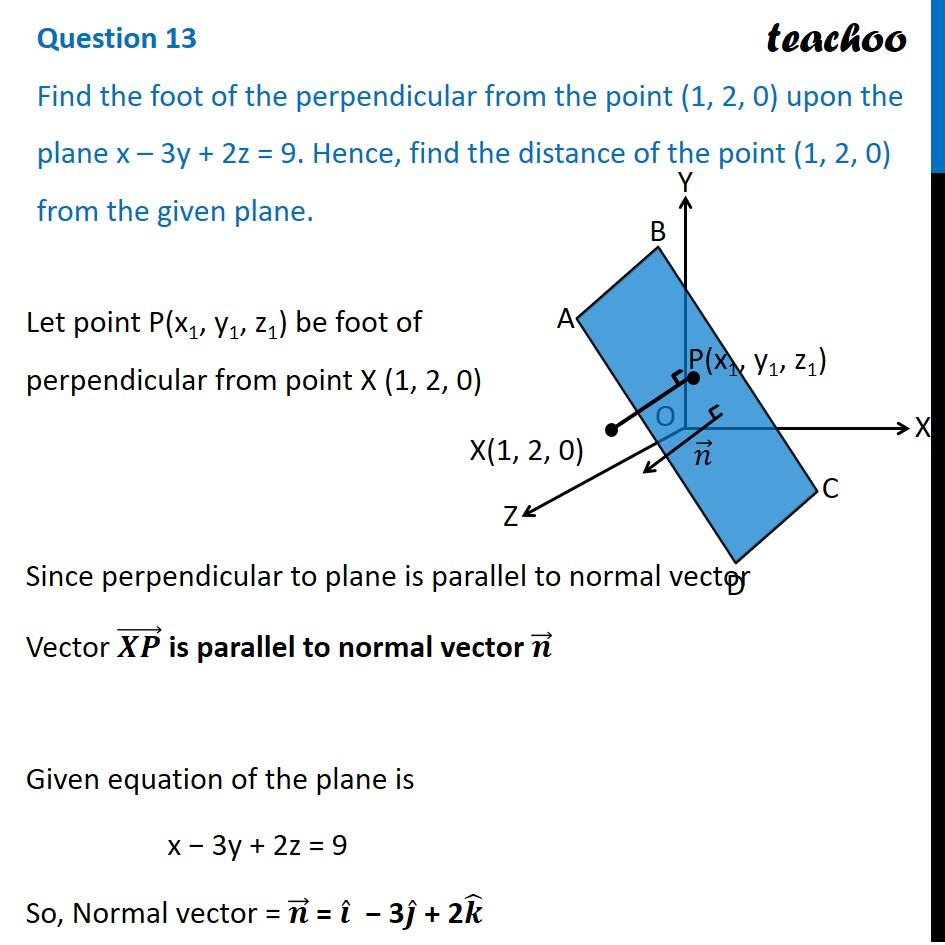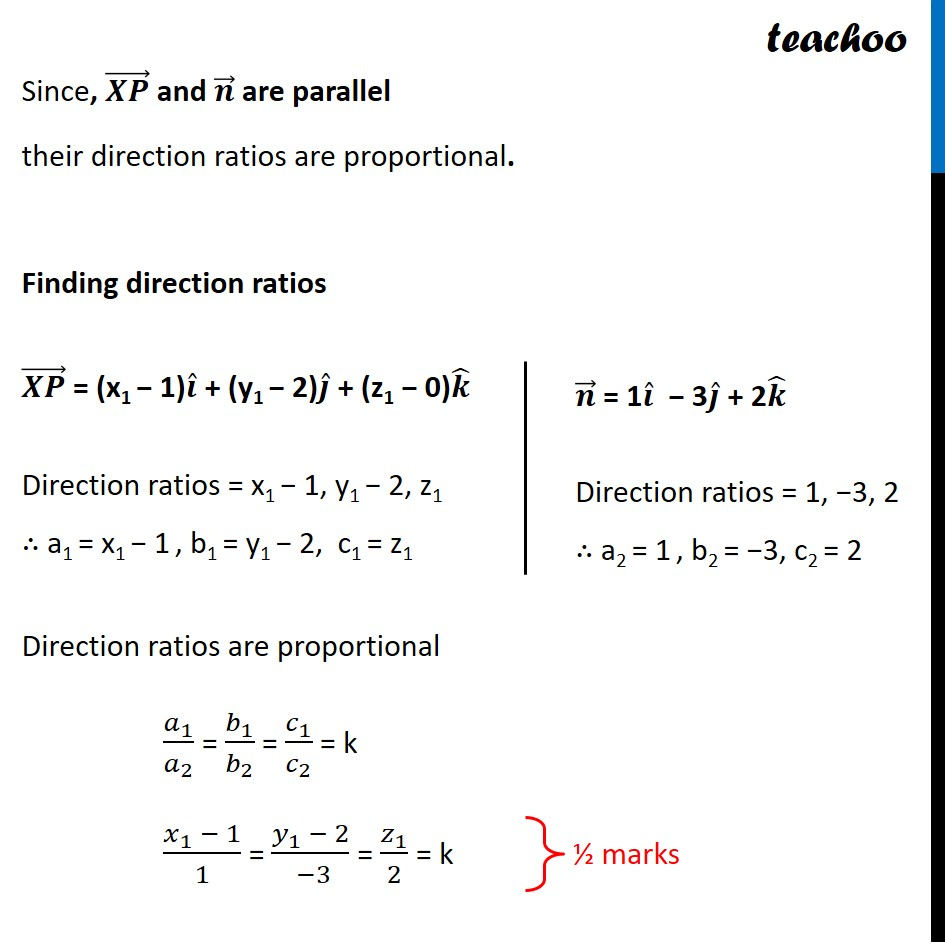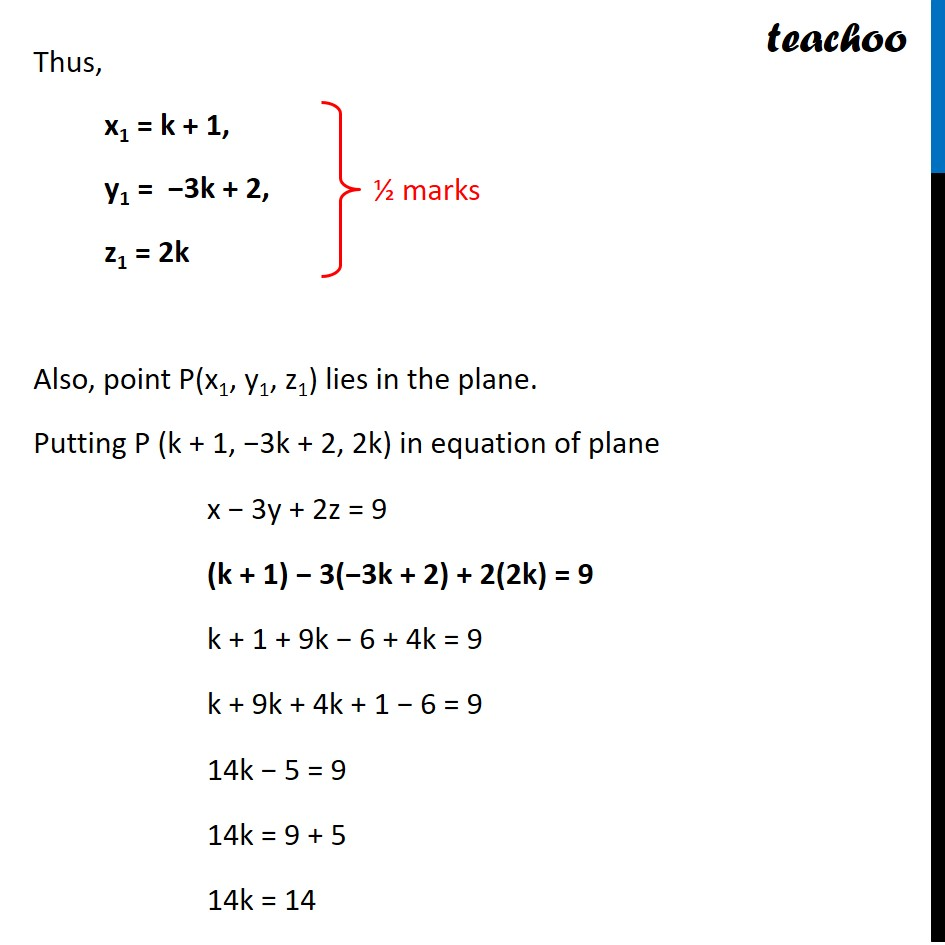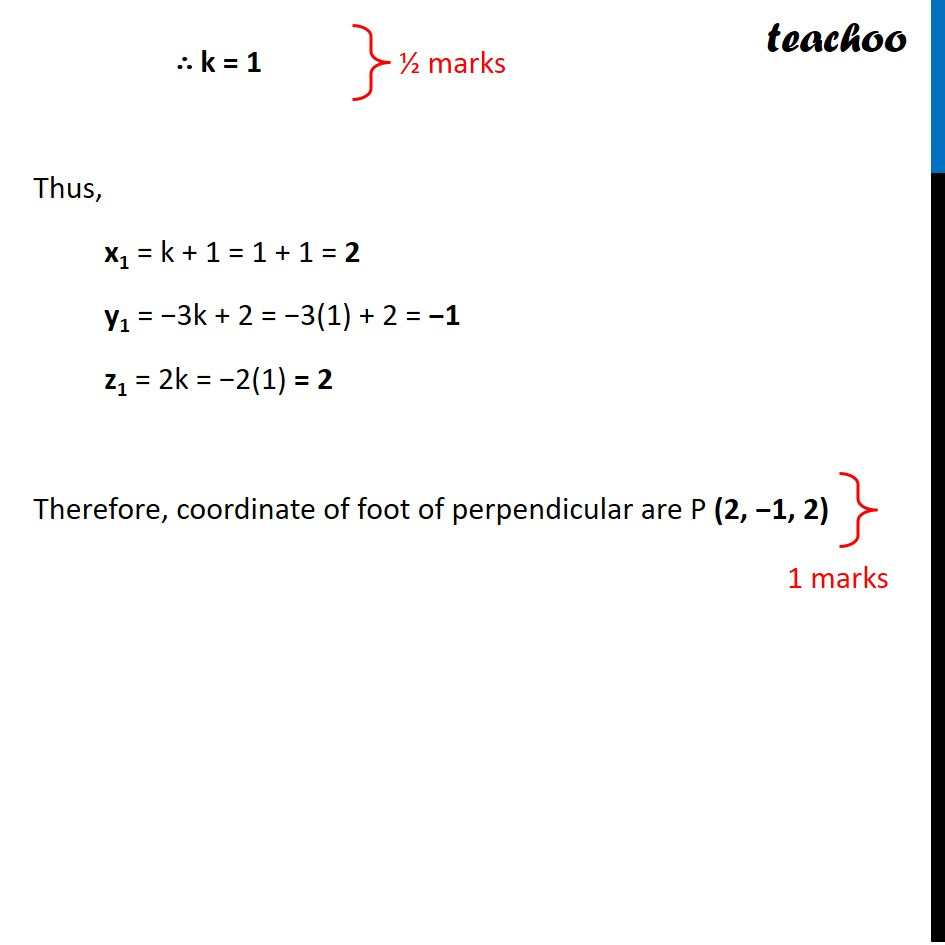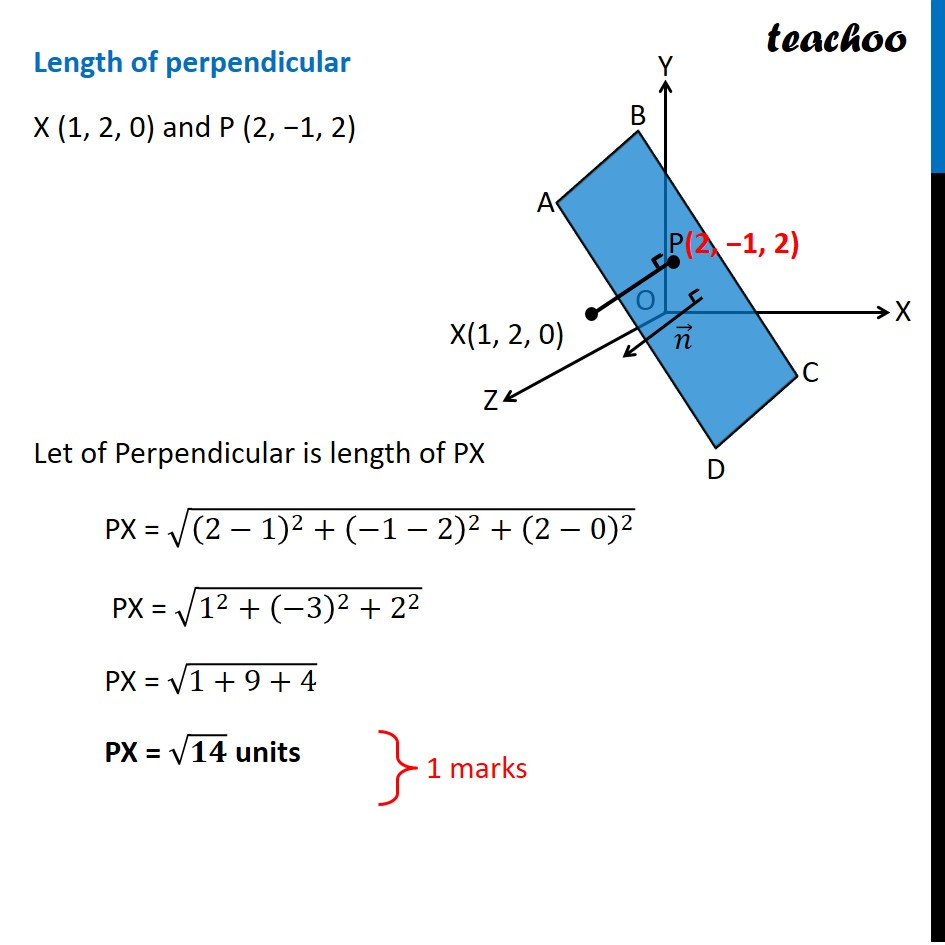Learn in your speed, with individual attention - Teachoo Maths 1-on-1 Class

### Transcript

Question 13 Find the foot of the perpendicular from the point (1, 2, 0) upon the plane x – 3y + 2z = 9. Hence, find the distance of the point (1, 2, 0) from the given plane. Let point P(x1, y1, z1) be foot of perpendicular from point X (1, 2, 0) Since perpendicular to plane is parallel to normal vector Vector (𝑿𝑷) ⃗ is parallel to normal vector 𝒏 ⃗ Given equation of the plane is x − 3y + 2z = 9 So, Normal vector = 𝒏 ⃗ = 𝒊 ̂ − 3𝒋 ̂ + 2𝒌 ̂ Since, (𝑿𝑷) ⃗ and 𝒏 ⃗ are parallel their direction ratios are proportional. Finding direction ratios (𝑿𝑷) ⃗ = (x1 − 1)𝒊 ̂ + (y1 − 2)𝒋 ̂ + (z1 − 0)𝒌 ̂ Direction ratios = x1 − 1, y1 − 2, z1 ∴ a1 = x1 − 1 , b1 = y1 − 2, c1 = z1 𝒏 ⃗ = 1𝒊 ̂ − 3𝒋 ̂ + 2𝒌 ̂ Direction ratios = 1, −3, 2 ∴ a2 = 1 , b2 = −3, c2 = 2 Since, (𝑿𝑷) ⃗ and 𝒏 ⃗ are parallel their direction ratios are proportional. Finding direction ratios Direction ratios are proportional 𝑎_1/𝑎_2 = 𝑏_1/𝑏_2 = 𝑐_1/𝑐_2 = k (𝑥_1 − 1)/1 = (𝑦_1 − 2)/( −3) = 𝑧_1/2 = k Thus, x1 = k + 1, y1 = −3k + 2, z1 = 2k Also, point P(x1, y1, z1) lies in the plane. Putting P (k + 1, −3k + 2, 2k) in equation of plane x − 3y + 2z = 9 (k + 1) − 3(−3k + 2) + 2(2k) = 9 k + 1 + 9k − 6 + 4k = 9 k + 9k + 4k + 1 − 6 = 9 14k − 5 = 9 14k = 9 + 5 14k = 14 ∴ k = 1 Thus, x1 = k + 1 = 1 + 1 = 2 y1 = −3k + 2 = −3(1) + 2 = −1 z1 = 2k = −2(1) = 2 Therefore, coordinate of foot of perpendicular are P (2, −1, 2) Length of perpendicular X (1, 2, 0) and P (2, −1, 2) Let of Perpendicular is length of PX PX = √((2−1)^2+(−1−2)^2+(2−0)^2 ) PX = √(1^2+(−3)^2+2^2 ) PX = √(1+9+4) PX = √𝟏𝟒 units